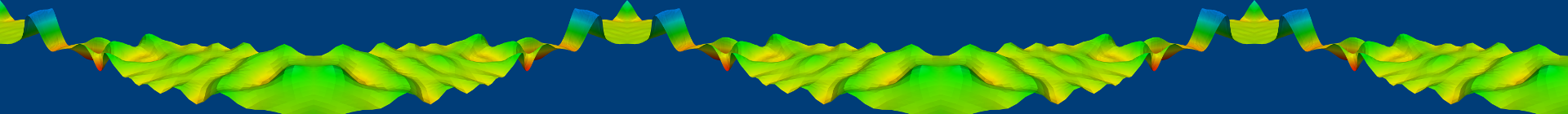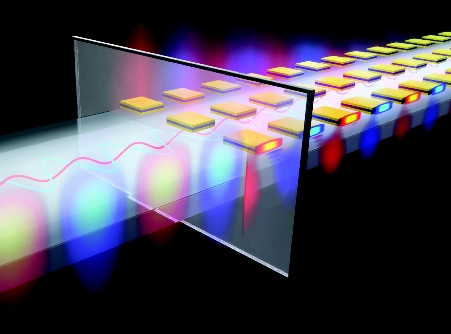# Project B4 • Effective characterization of optical metamaterials beyond a local response

### Principal investigators

 Prof. Dr. Michael Plum (7/2015 - ) Prof. Dr. Carsten Rockstuhl (7/2015 - )

### Project summaryFigure 1 Illustration of an interface between vacuum and an optical metamaterial made of cut-plate pairs.

In this project we aim to improve the description of light-matter interaction in optical metamaterials by going beyond local material laws. To be able to realistically discuss these artificial materials on physical grounds, we need to retain nonlocal effective material parameters. To this end, we rigorously derive (additional) interface conditions and study the dispersion relations.

## Introduction and Methodology

Optical metamaterials are usually made of periodically arranged building blocks called meta atoms. These materials show interesting effects that cannot be observed in natural media, e.g., a negative index behavior or an artificial magnetic response. To be able to discuss these effects on physical grounds, it is a prime challenge to introduce and assign effective material parameters to a given metamaterial by assuming that their corresponding parameters are spatially independent. This step is called homogenization in a physical sense.

Usually, local, effective material parameters are employed, but these turn out be insufficient to realistically describe the metamaterial in the operational wavelength regime. The description with local material parameters for example, fails to reproduce the electromagnetic response, i.e., the reflection and transmission from a slab metamaterial, at oblique incidence. The agreement with the actual response of a reference material is, therefore, limited to normal incidence. That is an intrinsic problem of local constitutive relations and not just a technical question to retrieve the correct material parameters. Unfortunately, the operational wavelength is not much longer than the typical size of the meta atom but only longer. The propagating light will probe to some extent for the details of the unit cell that render an effective description with local effective properties pointless. The meta atoms cannot be simply downscaled since the dispersive effects of interest would vanish.

Therefore, the goal of this project is to lift these limitations and to answer to the question, how can we improve the effective description of optical metamaterials, such that the macroscopic properties of the material are properly captured?

When the wavelength-to-unit cell ratio becomes close to one, spatial fluctuations of the fields become prominent. This also means that the light actually “sees” the geometry of the structure. It is therefore of utmost importance to introduce nonlocal, effective material properties, as the local description is not adequate in this regime. Nonlocality can be modeled as follows $D(r,\omega)=\int R(r-r',\omega)\,E(r',\omega)\,\text{d}r',$ where $D$ represents the induced electric displacement field, $R$ the response function of the material, and $E$ the electric field of light. The equation above shall be understood as follows. The electromagnetic response described by $D$ at a position $r$ is induced by an electric field $E$ at a distant position $r'$. This response is mediated by the response function $R$ that depends on both positions. Since the material is homogeneous, in the response function only $R$ depends on the relative positions of $r$ and $r'$, i.e., it only depends on the difference $r-r'$.

In this project we actively work with the nonlocal approach as defined above. The major task consists of finding nonlocal response functions $R$ where we can calculate the dispersion relation, i.e., solve the wave equation and derive the interface conditions of an interface between a local medium and a nonlocal, homogenized, metamaterial. To this end, we start from an approximate response function, written in Fourier space, given by the following Taylor approximation $R(k,\omega)=\varepsilon+k\times\alpha k\times-k\times k\times\gamma k\times k\times$ where $\varepsilon$, $\alpha$ and $\gamma$ are $3\times 3$ matrices depending om $\omega$ and represent the effective material parameters. By inverse Fourier transform, we can remount to differential operators. This formulation helps to derive interface conditions through the weak formulation of the obtained model.

## Results and Perspectives

With the inclusion of nonlocal effective material parameters, we managed to improve the current situation in terms of reproducing the reflection and the transmission coefficients from a metamaterial slab. We find that with the introduction of nonlocality we extend the applicability of homogenization beyond the paraxial regime up to an angle of incidence of 50°, compared to 20° previously, as shown in Figure 2. The results are obtained by means of least absolute deviations (least 1-norm) fits of the analytically derived reflection and transmission coefficients to the referential values for reflection and transmission of an actual metamaterial slab. The parameters that optimize the fitting, will be defined as the effective material parameters of the metamaterial. We use the Fourier Modal Method (FMM) for generating the reference data, that can be safely assumed (up to a numerical precision) to be exact. This methodology can be also applied in experimental setups in which reflection and transmission can be measured.Figure 2 Reflection (left) and transmission (right) coefficients as a function of the angle of incidence. The solid (dashed) curves represent the real (imaginary) part. The reference curves in black are numerically obtained from a plane wave expansion ansatz. The blue curves are obtained from the attempt of reproducing the black curves while considering the local approach and the red ones for the case of nonlocal approach.

We have shown that for a realistic effective description of optical metamaterials, it is important to consider nonlocal material laws. For further research, we aim to include higher-order nonlocality into response function $R$ to push the agreement for wavelength-to-unit cell ratios close to one and for even larger angles of incidences. This requires interdisciplinary work from both physical and mathematical sides.

## Publications

1. , , , , and . Towards more general constitutive relations for metamaterials: a checklist for consistent formulations. Phys. Rev. B, 101(19):195411, May . [preprint] [bibtex]

2. , , , and . Homogenization of wire media with a general purpose nonlocal constitutive relation. J. Opt. Soc. Am. B, 36(8):F99–F108, August . [bibtex]

3. , , , and . Retrieving effective material parameters of metamaterials characterized by nonlocal constitutive relations. Phys. Rev. B, 99(3):035442, January . [bibtex]

4. , , and . Exact multipolar decompositions with applications in nanophotonics. Adv. Opt. Mater., 7(1):1800783, January . [bibtex]

5. , , , , , and . Surface plasmon polaritons sustained at the interface of a nonlocal metamaterial. Phys. Rev. B, 98(11):115409, September . [bibtex]

6. , , , , and . Beyond local effective material properties for metamaterials. Phys. Rev. B, 97(7):075439, February . [preprint] [bibtex]

7. and . Spectrum of a singularly perturbed periodic thin waveguide. J. Math. Anal. Appl., 454(2):673–694, October . [preprint] [bibtex]

8. , , , and . Computing the T-matrix of a scattering object with multiple plane wave illuminations. Beilstein J. Nanotechnol., 8:614–626, March . [bibtex]

9. , , , and . Transverse multipolar light-matter couplings in evanescent waves. Phys. Rev. A, 94(5):053822, November . [preprint] [bibtex]

10. , , , and . Optically assisted trapping with high-permittivity dielectric rings: Towards optical aerosol filtration. Appl. Phys. Lett., 109(14):141102, October . [preprint] [bibtex]

11. , , , and . Exact dipolar moments of a localized electric current distribution. Opt. Express, 23(26):33044–33064, 12 . [preprint] [bibtex]

## Preprints

1. , , , , and . Higher order constitutive relations and interface conditions for metamaterials with strong spatial dispersion. CRC 1173 Preprint 2020/37, Karlsruhe Institute of Technology, December . [bibtex]

2. , , , , and . Interface conditions for a metamaterial with strong spatial dispersion. CRC 1173 Preprint 2017/23, Karlsruhe Institute of Technology, September . [bibtex]

## Theses

1. . Modeling optical metamaterials with strong spatial dispersion. PhD thesis, Karlsruhe Institute of Technology (KIT), November . [bibtex]

2. . Spectral and asymptotic properties of periodic media. Habilitation thesis, Karlsruhe Institute of Technology (KIT), January . [bibtex]

Former staff
Name Title Function
Dr. Doctoral researcher
Dr. Postdoctoral researcher
Dr. Postdoctoral researcher
Dr. Postdoctoral researcher
Dr. Postdoctoral researcher